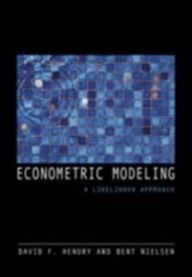# Econometric Modeling

Princeton University Press

• 380 kr

Kun 1 igjen

Econometric Modeling: A Likelihood Approach. Econometric Modeling provides a new and stimulating introduction to econometrics, focusing on modeling. The key issue confronting empirical economics is to establish sustainable relationships that are both supported by data and interpretable from economic theory. The unified likelihood-based approach of this book gives students the required statistical foundations of estimation and inference, and leads to a thorough understanding of econometric techniques. David Hendry and Bent Nielsen introduce modeling for a range of situations, including binary data sets, multiple regression, and cointegrated systems. In each setting, a statistical model is constructed to explain the observed variation in the data, with estimation and inference based on the likelihood function. Substantive issues are always addressed, showing how both statistical and economic assumptions can be tested and empirical results interpreted. Important empirical problems such as structural breaks, forecasting, and model selection are covered, and Monte Carlo simulation is explained and applied. Econometric Modeling is a self-contained introduction for advanced undergraduate or graduate students. Throughout, data illustrate and motivate the approach, and are available for computer-based teaching. Technical issues from probability theory and statistical theory are introduced only as needed. Nevertheless, the approach is rigorous, emphasizing the coherent formulation, estimation, and evaluation of econometric models relevant for empirical research.

 Forfattere: Bent Nielsen, David F. Hendry Utgave: ukjent Språk: Engelsk Sidetall: 365 ISBN: 9780691130897 Vekt: 794 g Forlag: Princeton University Press Innbinding: Heftet Utgitt: 2007 Veil. pris: 0 kr
Salg

Unavailable

Sold Out